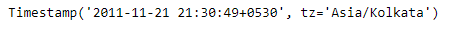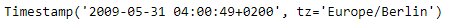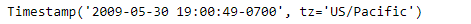# Python | Pandas Timestamp.tz_convert

Python is a great language for doing data analysis, primarily because of the fantastic ecosystem of data-centric python packages. Pandas is one of those packages and makes importing and analyzing data much easier.

Pandas` Timestamp.tz_convert()` function convert tz-aware Timestamp to another time zone. The function takes the desired timezone as an input to which we want to convert to.

Syntax :Timestamp.tz_convert()

Parameters :
tz : Time zone for time which Timestamp will be converted to. None will remove timezone holding UTC time.

Return : converted : Timestamp

Example #1: Use `Timestamp.tz_convert()` function to convert the given tz-aware Timestamp object to ‘Asia/Kolkata’.

 `# importing pandas as pd ` `import` `pandas as pd ` ` `  `# Create the Timestamp object ` `ts ``=` `pd.Timestamp(year ``=` `2011``,  month ``=` `11``, day ``=` `21``,  ` `                  ``hour ``=` `10``, second ``=` `49``, tz ``=` `'US/Central'``)  ` ` `  `# Print the Timestamp object ` `print``(ts) `

Output :Now we will use the `Timestamp.tz_convert()` function to convert the timezone of ts object to ‘Asia/Kolkata’.

 `# convert to 'Asia / Kolkata' ` `ts.tz_convert(tz ``=` `'Asia/Kolkata'``) `

Output :As we can see in the output, the `Timestamp.tz_convert()` function has converted the timezone of the given object to ‘Asia/Kolkata’.

Example #2: Use `Timestamp.tz_convert()` function to convert the given tz-aware Timestamp object to ‘US/Pacific’.

 `# importing pandas as pd ` `import` `pandas as pd ` ` `  `# Create the Timestamp object ` `ts ``=` `pd.Timestamp(year ``=` `2009``, month ``=` `5``, day ``=` `31``,  ` `                  ``hour ``=` `4``, second ``=` `49``, tz ``=` `'Europe/Berlin'``) ` ` `  `# Print the Timestamp object ` `print``(ts) `

Output :Now we will use the `Timestamp.tz_convert()` function to convert the timezone of ts object to ‘US/Pacific’.

 `# convert to 'US / Pacific' ` `ts.tz_convert(tz ``=` `'US/Pacific'``) `

Output :As we can see in the output, the `Timestamp.tz_convert()` function has converted the timezone of the given object to ‘US/Pacific’.

My Personal Notes arrow_drop_upCheck out this Author's contributed articles.

If you like GeeksforGeeks and would like to contribute, you can also write an article using contribute.geeksforgeeks.org or mail your article to contribute@geeksforgeeks.org. See your article appearing on the GeeksforGeeks main page and help other Geeks.

Please Improve this article if you find anything incorrect by clicking on the "Improve Article" button below.

Article Tags :

Be the First to upvote.

Please write to us at contribute@geeksforgeeks.org to report any issue with the above content.